Next: Subsonic Flow Past a Up: Two-Dimensional Compressible Inviscid Flow Previous: Homenergic Homentropic Flow

# Small-Perturbation Theory

A great number of problems of interest in compressible fluid mechanics are concerned with the perturbation of a known flow pattern. The most common case is that of uniform, steady flow. Letdenote the uniform flow velocity, which is directed parallel to the-axis. The density, pressure, and temperature are also assumed to be uniform, and are denoted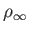,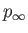, and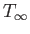, respectively. The corresponding sound speed is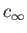, and the Mach number is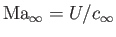. Finally. the velocity field of the unperturbed flow pattern is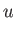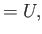(15.102)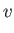(15.103)

Suppose that a solid body, such as an airfoil, is placed in the aforementioned flow pattern. The cross-section of the body is assumed to be independent of the Cartesian coordinate. The body disturbs the flow pattern, and changes its velocity field, which is now written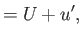(15.104)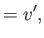(15.105)

where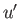and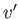are known as induced velocity components. We are interested in situations in which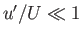and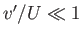.

Equation (15.101) can be combined with the previous two equations to give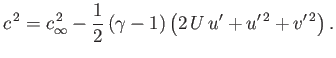(15.106)

It then follows from Equation (15.100) that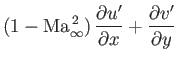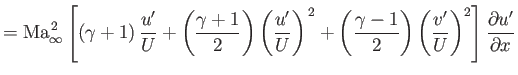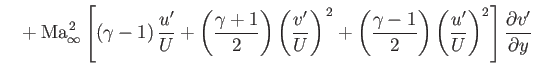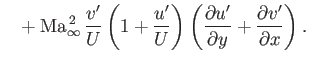(15.107)

The previous equation is exact. However, if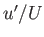and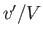are small then it becomes possible to neglect many of the terms on the right-hand side. For instance, neglecting terms that are third-order in small quantities, we obtain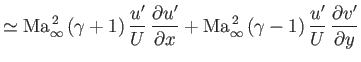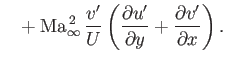(15.108)

Furthermore, if we neglect terms that are second-order in small quantities then we get the linear equation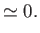(15.109)

Note, however, than in so-called transonic flow, where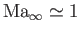, the coefficient of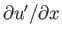on the left-hand side of Equation (15.108) becomes very small. In this situation, it is not possible to neglect the first term on the right-hand side. However, the conditiondoes not affect the term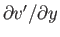on the left-hand side of Equation (15.108), and so the other terms on the right-hand side can still be neglected. Thus, transonic flow is governed by the non-linear equation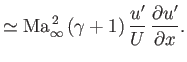(15.110)

On the other hand, subsonic (i.e.,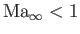) and supersonic flow (i.e.,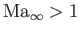) are both governed by Equation (15.109). Another situation in which certain terms on the right-hand side of Equation (15.108) must be retained is hypersonic flow (i.e.,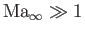). This follows because, althoughandare small, their products with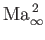can still be non-negligible. Roughly speaking, Equation (15.109) is valid for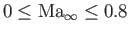and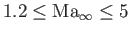. In other words, transonic flow corresponds to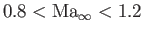, and hypersonic flow to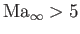(Anderson 2003).

The pressure coefficient is defined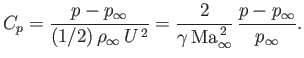(15.111)

(See Section 15.9.) Equation (15.101) implies that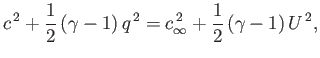(15.112)

where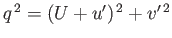. Moreover, Equation (14.61) yields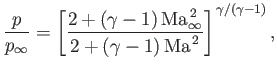(15.113)

where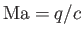. The previous two equations can be combined to give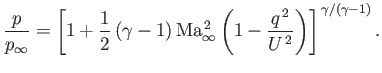(15.114)

Hence, we obtain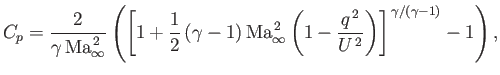(15.115)

which reduces to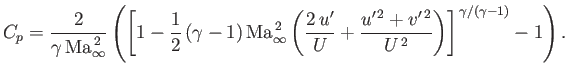(15.116)

Using the binomial expansion on the expression in square brackets, and neglecting terms that are third-order, or higher, in small quantities, we obtain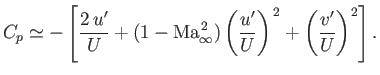(15.117)

For two-dimensional flows, in the limit in which Equation (15.109) is valid, it is consistent to retain only first-order terms in the previous equation, so that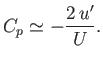(15.118)

Let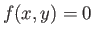(15.119)

be the equation of the surface of the solid body that perturbs the flow. At the surface, the velocity vector of the flow must be perpendicular to the local normal: that is, the flow must be tangential to the surface. In other words,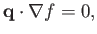(15.120)

which reduces to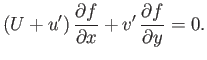(15.121)

Neglectingwith respect to, we obtain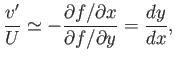(15.122)

where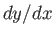is the slope of the surface, and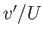the approximate slope of a streamline.

Now, the body has to be thin in order to satisfy our assumption that the induced velocities are relatively small. This implies that the coordinatediffers little from zero (say) on the surface of the body. Hence, we can write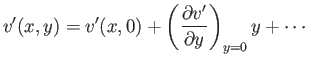(15.123)

in the immediate vicinity of the surface. Within the framework of small-perturbation theory, it is consistent to neglect all terms on the right-hand side of the previous equation after the first. Hence, the boundary condition (15.122) reduces to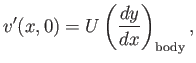(15.124)

respectively.

Because a homenergic, homentropic flow pattern is necessarily irrotational (see Section 15.10), we can write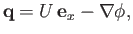(15.125)

where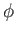is the perturbed velocity potential. (See Section 4.15.) It follows that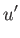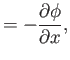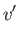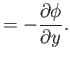(15.126)

Hence, Equations (15.109), (15.118), and (15.124) become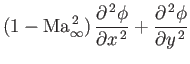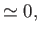(15.127)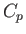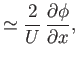(15.128)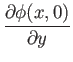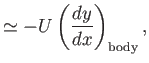(15.129)

respectively.Next: Subsonic Flow Past a Up: Two-Dimensional Compressible Inviscid Flow Previous: Homenergic Homentropic Flow
Richard Fitzpatrick 2016-03-31